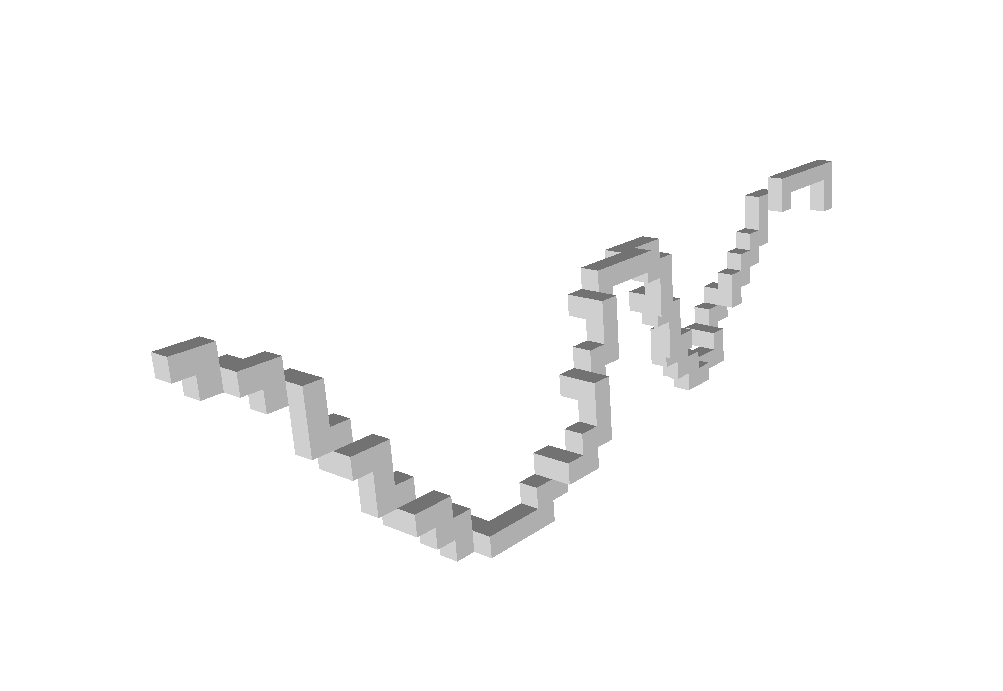DGtal  1.4.beta
geometry/curves/exampleGridCurve3d.cpp

This example shows how to use GridCurve as a sequence of 1-scells in a 3d Khalimsky space.

$./examples/geometry/curves/exampleGridCurve3d  Note that the data type you want to display may be passed as argument as follows: $ ./examples/geometry/curves/exampleGridCurve3d points


This command line produces the following output (points are displayed as voxels):3d digital curve
Analysis of one-dimensional discrete structures
#include <iostream>
#include "DGtal/base/Common.h"
#include "DGtal/helpers/StdDefs.h"
#include "ConfigExamples.h"
#include "DGtal/io/viewers/Viewer3D.h"
#include "DGtal/geometry/curves/GridCurve.h"
#ifdef WITH_VISU3D_QGLVIEWER
#include "DGtal/io/DrawWithDisplay3DModifier.h"
#endif
using namespace std;
using namespace DGtal;
using namespace Z3i;
int main( int argc, char** argv )
{
trace.info() << "exampleGridCurve3d: the type of data to be displayed "
<< "may be given as argument as follows: "
<< argv << " scells" << endl;
trace.info() << "Available types are: gridcurve (default), scells, points, midpoints, arrows" << endl;
string type = (argc > 1) ? string(argv) : "gridcurve";
trace.info() << "Chosen type: " << type << endl;
//curve
string sinus = examplesPath + "samples/sinus.dat";
// domain
Point lowerBound = Point::diagonal( -100 );
Point upperBound = Point::diagonal( 100 );
K3 ks; ks.init( lowerBound, upperBound, true );
GridCurve<K3> gc( ks );
fstream inputStream;
inputStream.open (sinus.c_str(), ios::in);
gc.initFromVectorStream(inputStream);
inputStream.close();
bool flag = false;
#ifdef WITH_VISU3D_QGLVIEWER
QApplication application(argc,argv);
Viewer3D<Space,K3> viewer(ks);
viewer.show();
if (type == "gridcurve")
{
viewer << gc;
}
else if (type == "scells")
{
viewer << gc.getSCellsRange();
}
else if (type == "points")
{
viewer << gc.getPointsRange();
}
else if (type == "midpoints")
{
viewer << gc.getMidPointsRange();
}
else if (type == "arrows")
{
viewer << gc.getArrowsRange();
}
else
{
trace.info() << "Display type not known." << std::endl;
}
viewer << Viewer3D<Space,K3>::updateDisplay;
flag = application.exec();
#endif
return flag;
}
// //
bool init(const Point &lower, const Point &upper, bool isClosed)
Specifies the upper and lower bounds for the maximal cells in this space.
std::ostream & info()
KSpace K3
Definition: StdDefs.h:147
DGtal is the top-level namespace which contains all DGtal functions and types.
Trace trace
Definition: Common.h:153
int main(int argc, char **argv)
MyPointD Point
Definition: testClone2.cpp:383